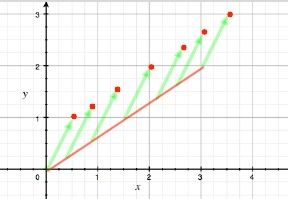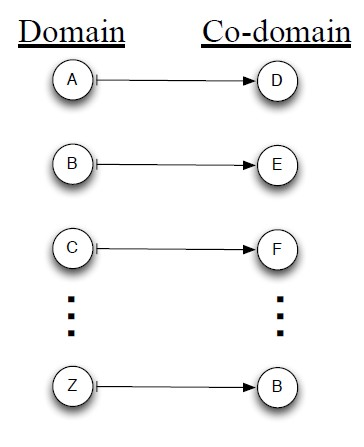# Linear Algebra - Vector Space (set of vector)

## Definition

A vector space is a subset of the set of function $F^D$ representing a geometric object passing through the origin.

A vector space over a field F is any set V of vector :

• with the addition and scalar-multiplication operation
• satisfying certain axioms (e.g. commutate and distributive laws)
• and the following properties P1, P2, P3.

This geometric subset of $F^D$ satisfies three properties:

• P1: They contains the zero vector 0 (The origin). A vector space always contains the zero vector.
• P2: If subset contains v then it contains $\alpha.v$ for every scalar $\alpha$
• P3: If subset contains u and v then it contains u + v

The image of a linear function $f : V \rightarrow W$ is a vector space.

## Representation

There is different way to specify a vector space:

There is two natural way (Dual Representation) to specify a vector space V (of every subspace of $\mathbb{R}^D$ ). It's to specify a basis in terms:

### generator

of generators for V. $$V = Span \{v_1, \dots , v_n\}$$

Matrix equivalent to: $$V = Row \begin{bmatrix} \begin{array}{r} v_1 \\ \hline \\ \vdots \\ \hline \\ v_n \end{array} \end{bmatrix}$$

Computational Problem: Finding a basis of the vector space spanned by given vectors:

• input: a list [v1, . . . , vn] of vectors
• output: a list of vectors that form a basis for Span {v1, . . . , vn}.

### homogeneous linear system

or of a homogeneous linear system whose solution set is the vector space V. $$V = \text{Solution set of homogeneous linear system} \{x : a_1.x = 0, \dots, a_m.x = 0\}$$

matrix equivalent to:

$$V = \href{matrix#null space}{Null} \begin{bmatrix} \begin{array}{r} a_1 \\ \hline \\ \vdots \\ \hline \\ a_n \end{array} \end{bmatrix}$$

Computational Problem: Finding a basis of the solution set of a homogeneous linear system

• input: a list [a1, . . . , an] of vectors
• output: a list of vectors that form a basis for the set of solutions to the system a1 · x = 0, . . . , an · x = 0

## Operations

### Representation Transformation

#### From generators to homogeneous system

Definition:

• Given system a1 · x = 0, . . . , am · x = 0, find generators v1, . . . , vn for solution set
• Equivalently, given matrix A, find B such that Row B = Null A

Solution set is:

• x, the set of vectors u such that a1 · u = 0, . . . , am · u = 0

$$\underbrace{ \begin{bmatrix} \begin{array}{r} a_1 \\ \hline \\ \vdots \\ \hline \\ a_n \end{array} \end{bmatrix}}_{A} \begin{bmatrix} \begin{array}{r} \\ \\ x \\ \\ \end{array} \end{bmatrix} = \begin{bmatrix} \begin{array}{r} 0 \\ \hline \\ \vdots \\ \hline \\ 0 \end{array} \end{bmatrix}$$

• Equivalent: Given rows of a matrix A, find generators for Null A

If u is such a vector then $$u · (\alpha_1.a_1 + \dots + \alpha_m.a_m) = 0$$ for any coefficient $\alpha_1, \dots, \alpha_m$

Two equiavalent computations where Algorithm X solves this operation:

• rows of a matrix A → Algorithm X → generators for Null A
• generators for a vector space V → Algorithm X → generators for dual space V*

#### From homogeneous system to generators

Definition

• Given generators v1, . . . , vn, find system a1 · x = 0, . . . , am · x = 0 whose solution set equals Span {v1, . . . , vn}
• Equivalently, given matrix B, find matrix A such that Null A = Row B

Computation:

• generators for dual space V* → Algorithm Y → generators for original space V

A (V*)* = V. The dual of the dual is the original space. Algorithm X = Algorithm Y

## Lexique

### Span

The set of all linear combinations of some vectors v1,…,vn is called the span of these vectors and contains always the origin.

### Generator

The generators for the set of vectors $V$ are the vectors $v_1, \dots,v_n$ in the following formula:

$$V = Span \{v_1,\dots,v_n\}$$

where $\{v_1,\dots,v_n\}$ is a generating set for $V$

### Dimension

The dimension of a vector space is the size of a basis for that vector space. The dimension of a vector space V is written dim V.

### Basis

Lemma: Every finite set T of vectors contains a subset S that is a basis for Span T.

## Type

### Affine

If c is a vector and $V$ is a vector space then

$c + V$

is called an affine space

Example: A plane or a line not necessarily that contain the origin

### Subspace

Let $\upsilon$ and $\gamma$ be a vector space, if $\upsilon$ is a subset of $\gamma$ then $\upsilon$ is called a subspace of $\gamma$ .

Dimension Lemma: If U is a subspace of W then:

• Property D1: dim U <= dimW, and
• Property D2: if dim U = dimW then U = W

### Complementary subspace

When $U \href{direct_sum}{\oplus} V = W$ , U and V are complementary subspace of W.

Example: Suppose U is a plane in $\mathbb{R}^3$ . Then any line through the origin that does not lie in U is complementary subspace with respect to $\mathbb{R}^3$

For any finite-dimensional vector space W and any subspace U, there is a subspace V such that U and V are complementary.

### Orthogonal complement

Let U be a subspace of W. For each vector b in W, we can write b as the following projections]]: $$b = b^{||U} + b^{\perp U}$$ where:

• $b^{||U}$ is in U, and
• $b^{\perp U}$ is orthogonal to every vector in U.

Let V be the set $\{b^{\perp U} : b \in W\}$ . V is the orthogonal complement of U in W

Recommended PagesFunction - Scalar

Scalar functions return a single value (as opposed to an array). Mathematics definition: Any function whose domain is a vector space and whose value is its scalar field. Is the result of a scalar function...Linear Algebra

“Linear” “algebra” is the branch of mathematics: concerning vector spaces, often finite or countably infinite dimensional, as well as linear mappings between such spaces. Such an investigation...Linear Algebra - (Direct Sum | Union) of vector spaces

Let U and V be two vector spaces consisting of D-vectors over a field F. Definition: If U and V share only the zero vector then we define the direct sum of U and V to be the set: written: That is, ...Linear Algebra - (Linear system|System of Linear equations)

In mathematics, a system of linear equations (or linear system) is a collection of linear equations involving the same set of variables. Each linear system corresponds to a linear system with zero...Linear Algebra - (Line|Line Segment)

in linear algebra. Line and line segment definition in Linear Algebra that goes: A line has a dimension of one because only one coordinate is needed to specify a point on it Line_segmentline...Linear Algebra - Basis of a Vector Space

A basis for vector space V is a linearly independent set of generators for V. Thus a set S of vectors of V is a basis for V if S satisfies two properties: Property B1 (Spanning) Span S = V, and Property...Linear Algebra - Closest point in higher dimension than a plane

Solving closest point in the span of many vectors Goal: An algorithm that, given a vector b and vectors v1, . . . , vn, finds the vector in Span {v1, . . . , vn} that is closest to b. Special case:...Linear Algebra - Coordinate system

coordinate system in terms of vector. Idea of coordinate system for a vector space V: (and generalized beyond two dimensions), Coordinate system for a vector space V is specified by generators of...Linear Algebra - Function (Set)

For each input element in a set , a function assigns a single output element from another set . is called the domain of the function is called the co-domain or in Mathese: The function...Linear Algebra - Generators of a Vector Space

The generators for the set of vectors are the vectors in the following formula: where is a generating set for {[3, 0, 0], [0, 2, 0], [0, 0, 1]} is a generating set for . = Span {[3, 0,...